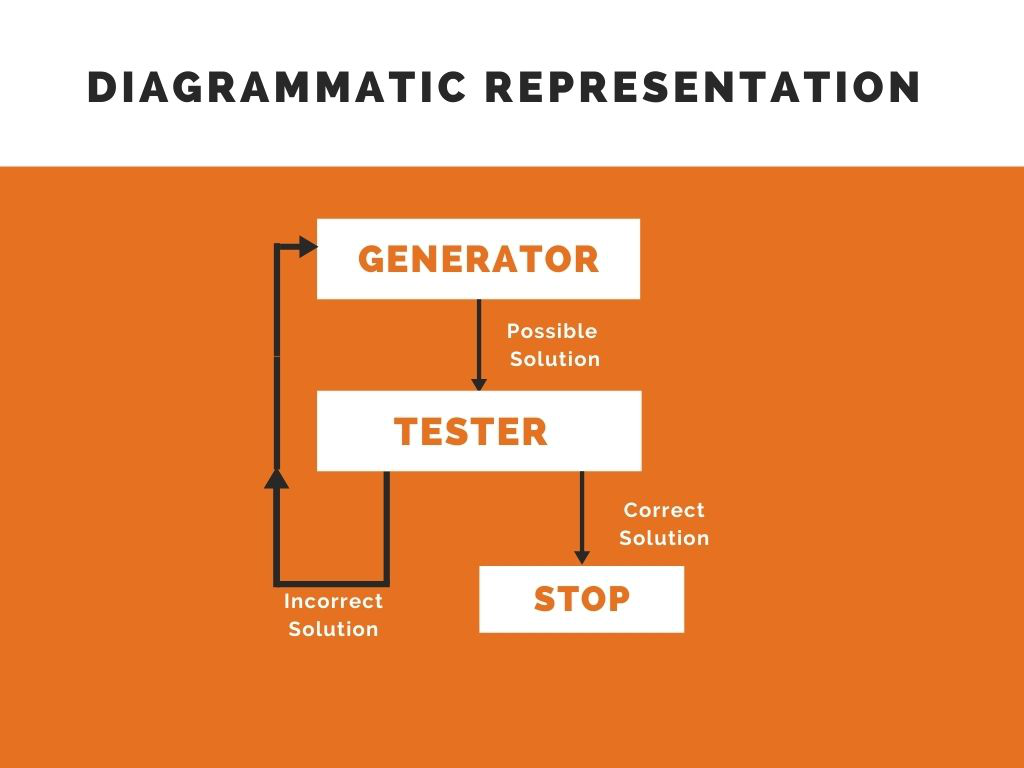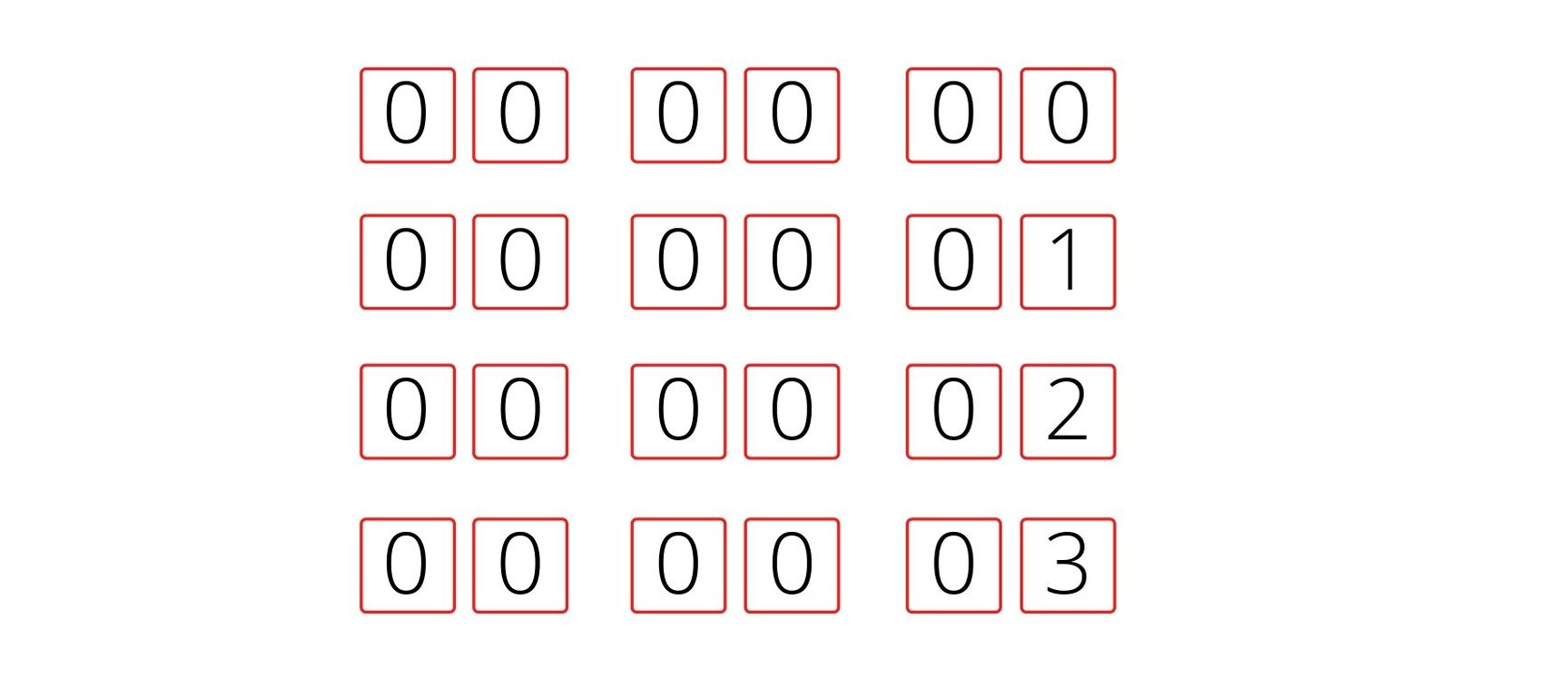Skip to content
Related Articles
Generate and Test Search
• Difficulty Level : Medium
• Last Updated : 22 Jan, 2021

### Introduction:

Generate and Test Search is a heuristic search technique based on Depth First Search with Backtracking which guarantees to find a solution if done systematically and there exists a solution. In this technique, all the solutions are generated and tested for the best solution. It ensures that the best solution is checked against all possible generated solutions.

It is also known as British Museum Search Algorithm as it’s like looking for an exhibit at random or finding an object in the British Museum by wandering randomly.

The evaluation is carried out by the heuristic function as all the solutions are generated systematically in generate and test algorithm but if there are some paths which are most unlikely to lead us to result then they are not considered. The heuristic does this by ranking all the alternatives and is often effective in doing so. Systematic Generate and Test may prove to be ineffective while solving complex problems. But there is a technique to improve in complex cases as well by combining generate and test search with other techniques so as to reduce the search space. For example in Artificial Intelligence Program DENDRAL we make use of two techniques, the first one is Constraint Satisfaction Techniques followed by Generate and Test Procedure to work on reduced search space i.e. yield an effective result by working on a lesser number of lists generated in the very first step.

### Algorithm

1. Generate a possible solution. For example, generating a particular point in the problem space or generating a path for a start state.
2. Test to see if this is a actual solution by comparing the chosen point or the endpoint of the chosen path to the set of acceptable goal states
3. If a solution is found, quit. Otherwise go to Step 1### Properties of Good Generators:

The good generators need to have the following properties:

• Complete:  Good Generators need to be complete i.e. they should generate all the possible solutions and cover all the possible states. In this way, we can guaranty our algorithm to converge to the correct solution at some point in time.
• Non Redundant:  Good Generators should not yield a duplicate solution at any point of time as it reduces the efficiency of algorithm thereby increasing the time of search and making the time complexity exponential. In fact, it is often said that if solutions appear several times in the depth-first search then it is better to modify the procedure to traverse a graph rather than a tree.
• Informed: Good Generators have the knowledge about the search space which they maintain in the form of an array of knowledge. This can be used to search how far the agent is from the goal, calculate the path cost and even find a way to reach the goal.

Let us take a simple example to understand the importance of a good generator. Consider a pin made up of three 2 digit numbers i.e. the numbers are of the form,In this case, one way to find the required pin is to generate all the solutions in a brute force manner for example,The total number of solutions in this case is (100) which is approximately 1M. So if we do not make use of any informed search technique then it results in exponential time complexity. Now let’s say if we generate 5 solutions every minute. Then the total numbers generated in 1 hour are 5*60=300 and the total number of solutions to be generated are 1M. Let us consider the brute force search technique for example linear search whose average time complexity is N/2. Then on an average, the total number of the solutions to be generated are approximately 5 lakhs. Using this technique even if you work for about 24 hrs a day then also you will need 10 weeks to complete the task.

Now consider using heuristic function where we have domain knowledge that every number is a prime number between 0-99 then the possible number of solutions are (25)3 which is approximately 15,000. Now consider the same case that you are generating 5 solutions every minute and working for 24 hrs then you can find the solution in less than 2 days which was being done in 10 weeks in the case of uninformed search.

We can conclude for here that if we can find a good heuristic then time complexity can be reduced gradually. But in the worst-case time and space complexity will be exponential. It all depends on the generator i.e. better the generator lesser is the time complexity.

### Interesting Real Life Examples:

• If a sufficient number of monkeys were placed in front of a set of typewriters, and left alone long enough, then they would eventually produce all the works of Shakespeare.
• Dendral which infers the structure of organic compounds using NMR spectrogram also uses plan-generate-test.My Personal Notes arrow_drop_up